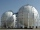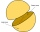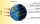# Expression of a variable from the formula + spherical cap - math problems

#### Number of problems found: 17

• Spherical cap 4What is the surface area of a spherical cap, the base diameter 20 m, height 2.5 m? Calculate using formula.
• Spherical capThe spherical cap has a base radius of 8 cm and a height of 5 cm. Calculate the radius of a sphere of which this spherical cap is cut.
• Spherical capPlace a part of the sphere on a 4.6 cm cylinder so that the surface of this section is 20 cm2. Determine the radius r of the sphere from which the spherical cap was cut.
• Spherical segmentThe spherical segment with height h=1 has a volume V=223. Calculate the radius of the sphere of which is cut this segment.
• Spherical section cutFind the volume of a spherical section if the radius of its base is 10 cm and the magnitude of the central angle ω = 120 degrees.
• Spherical segmentCalculate the volume of a spherical segment 18 cm high. The diameter of the lower base is 80 cm, the upper base 60 cm.
• Hemisphere cutCalculate the spherical layer's volume that remains from the hemisphere after the 3 cm section is cut. The height of the hemisphere is 10 cm.
• Spherical tankThe tank of a water tower is a sphere of radius 35ft. If the tank is filled to one quarter of full, what is the height of the water?
• PilotHow high is the airplane's pilot to see 0.001 of Earth's surface?
• AirplaneAviator sees part of the earth's surface with an area of 200,000 square kilometers. How high he flies?
• Above EarthTo what height must a boy be raised above the earth to see one-fifth of its surface.
• Sphere cutA sphere segment is cut off from a sphere k with radius r = 1. The volume of the sphere inscribed in this segment is equal to 1/6 of the segment's volume. What is the distance of the cutting plane from the center of the sphere?
• Two hemispheresIn a wooden hemisphere with a radius r = 1, a hemispherical depression with a radius r/2 was created so that the bases of both hemispheres lie in the same plane. What is the surface of the created body (including the surface of the depression)?
• The observatoryThe dome of the hemisphere-shaped observatory is 5.4 meters high. How many square meters of sheet metal needs to be covered to cover it, and 15 percent must be added to the minimum amount due to joints and waste?
• Tropics and polar zonesWhat percentage of the Earth's surface lies in the tropical, temperate, and polar zone? Individual zones are bordered by tropics 23°27' and polar circles 66°33'.
• Sphere parts, segmentA sphere with a diameter of 20.6 cm, the cut is a circle with a diameter of 16.2 cm. .What are the volume of the segment and the surface of the segment?
• MO SK/CZ Z9–I–3John had the ball that rolled into the pool, and it swam in the water. Its highest point was 2 cm above the surface. The diameter of the circle that marked the water level on the surface of the ball was 8 cm. Find the diameter of John ball.

Do you have an interesting mathematical word problem that you can't solve it? Submit a math problem, and we can try to solve it.

We will send a solution to your e-mail address. Solved examples are also published here. Please enter the e-mail correctly and check whether you don't have a full mailbox.

Please do not submit problems from current active competitions such as Mathematical Olympiad, correspondence seminars etc...

Expression of a variable from the formula - math problems. Spherical cap Problems.